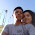## How to

When solving these problems, learning by doing is much better than learning by reading. I encourage to you read only as far in the solution as you need, then trying to solve the problem. If you get stuck, try reading a little further. And of course, let me know if you find a better solution!

## Thursday, February 9, 2012

### Compare Two Binary Trees

Solution:  Given two binary trees, return true if they are structurally identical.
int compareTrees(struct node * tree1, struct node * tree2) {
...
}

Let's start solving this one by drawing a picture of the trees to make sure that we fully get the problem:

In pic 1.  We have two identical trees, both in terms of structure and values.  So, in this case, we would return true.

Now, let's think about how trees could be different by drawing out a few more trees, as in pic 2.  Here, we have three trees that are all different.
- tree 2 is different from tree1 by having a different value (10 instead of 8), and
- tree 3 is different from tree 1 by having a different structure (and additional node with 10)

So, our algorithm needs to ensure that we can distinguish for both of these cases.

Now, let's start thinking about an algorithm, since we have a good idea of the examples.

Since we need to determine if the trees are identical, at a minimum, we're going to need to compare every node in the trees (as we can't say for certain that they're the same until we have done that).  And, upon finding one difference, we can immediately stop.

Let's think of how we would do this intuitively.  Let's start by doing this with pic1, where we have two of the same trees.  We can start at the top node, and then look at each lower level, left to right and say, are these nodes equivalent in terms of: 1) their values, and 2) having the same ancestor structure.  This algorithm is intuitive, and also should work.  It is essentially a breadth-first search of each tree, comparing the nodes as we go.

Alternatively, we could try a depth first search, comparing the two nodes, which would also work.

Now, let's think about implementing the solution.  At first, it may be tempting to think about using functions like a pop, where we could pop nodes off of each tree and compare them, and return false if they are not equivalent, and true if we get to the common ends.  This would work, but let's say that it is clearly an inelegant solution, and assume that it's not allowed.

Given that this is a tree solution, it screams for recursion.  And, we already know about breadth-first and depth-first searching (worth reading up on).  In the recursive case, let's think about the base case, where we divide the tree into smaller and smaller sub-trees.  Eventually, we get to the point where the trees are both simply null nodes, which is the true case.  Then, the algorithm starts to come about by continuing to get to this case:

Let's take a look at a depth first algorithm:
- If we reach null for nodes, then the subtrees are 'true.'
- Otherwise, they're true if: values are the same, and the left tree and right tree are the same
- Otherwise they're false.

Now, coded up:

int compareTrees(struct node * tree1, struct node * tree2) {
//Recursive base case.  We could obviously not compare to
//null, but it makes readibility harder

if(tree1==null && tree2==null) return(true);

//Otherwise, lets return the result of their values being
//true and the sub-trees being identical when both are not
//empty

else if (tree1 !=null && tree2 !=null) {
return(tree1->value == tree2->value &&
compareTrees(tree1->left, tree2->left) &&
compareTrees(tree1->right, tree2->right)

);

}
//one is null and one is not, so they're not the same.
else return(false);
}

Amazing, two lines of code for what seemed pretty challenging.

Let's check our sample trees in pics 1 and 2.

Yup, it works for those.  Now, let's think about base cases.  If one tree is null, or either node is null, we catch that immediately in the first line, so there is no concern of having a tree of null, or of de-referencing a null pointer.

And, for run-time efficiency, in the worst case, this requires looking at every node, so it is O(n).  There is some recursive overhead too, which is worth mentioning to the interviewer.

Please let us know if you find bugs in this solution, have alternative solutions or other ways to solve this problem!

1.binary possibilities, as well as electronic digital possibilities while they're also known as, usually are investment decision possibilities that usually offer big earnings. You'll find investors that have come to be millionaires dealing most of these set as well as telephone possibilities.

2.I am having two binary trees T1 ,T2 with a(Root)-------b(Left)....c(Right Node)....but T1-- b node having one leaf and T2--b node having two leafs How these too Trees are equal plz explain

3.Holly God! Thank you for such a wonderful and useful explanation

4.You there, this is really good post here. Thanks for taking the time to post such valuable information. Quality content is what always gets the visitors coming. product comparison

5.Hello, this weekend is good for me, since this time i am reading this enormous informative article here at my home. Joel's Pro Tree Service

6.This is my first time visit to your blog and I am very interested in the articles that you serve. Provide enough knowledge for me. Thank you for sharing useful and don't forget, keep sharing useful info: Beavercreek